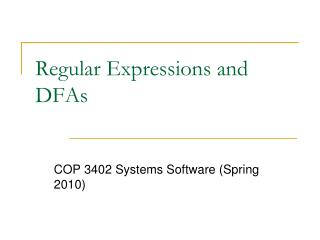Download PresentationRegular Expressions and DFAs

# Regular Expressions and DFAs

Download Presentation## Regular Expressions and DFAs

- - - - - - - - - - - - - - - - - - - - - - - - - - - E N D - - - - - - - - - - - - - - - - - - - - - - - - - - -
##### Presentation Transcript

1. Regular Expressions and DFAs COP 3402 Systems Software (Spring 2010)

2. Regular Expression • Notation to specify a set of strings

3. Examples

4. Exercise 1 • Let ∑ be a finite set of symbols • ∑ = {10, 11}, ∑* = ?

5. Answer • Answer: ∑* = {є, 10, 11, 1010, 1011, 1110, • 1111, …}

6. Exercises 2 • Let ∑ be a finite set of symbols and let L, L1, and L2 be sets of strings from ∑*. L1L2 is the set {xy | x is in L1, and y is in L2} • L1 = {10, 1}, L2 = {011, 11}, L1L2 = ?

7. Answer • L1L2 = {10011, 1011, 111}

8. Exercises 3 • Write RE for • All strings of 0’s and 1’s • All strings of 0’s and 1’s with at least 2  consecutive 0’s • All strings of 0’s and 1’s beginning with 1 and not having two consecutive 0’s

9. Answer • (0+1)* All strings of 0’s and 1’s • (0+1)*00(0+1)* All strings of 0’s and 1’s with at least 2  consecutive 0’s • (1+10)* (1+10) All strings of 0’s and 1’s beginning with 1  and not having two consecutive 0’s

10. More Exercises • 1) (0+1)*011 • 2) 0*1*2* • 3) 00*11*22*

11. More Exercises (Answers) 1) (0+1)*011 Answer: all strings of 0’s and 1’s ending in 011 2) 0*1*2* • Answer: any number of 0’s followed by  any number of 1’s followed by any number  of 2’s • 3) 00*11*22* Answer: strings in 0*1*2 with at least one of  each  symbol

12. Using Regular Expressions • Regular expressions are a standard programmer's tool. • Built in to Java, Perl, Unix, Python, . . . .

13. Deterministic Finite Automata (DFA) • Simple machine with N states. • Begin in start state. • Read first input symbol. • Move to new state, depending on current state and input symbol. • Repeat until last input symbol read. • Accept or reject string depending on label of last state.

14. DFA

15. Theory of DFAs and REs • RE. Concise way to describe a set of strings. • DFA. Machine to recognize whether a given string is in a given set. • Duality: for any DFA, there exists a regular expression to describe the same set of strings; for any regular expression, there exists a DFA that recognizes the same set.

16. Duality Example • DFA for multiple of 3 b’s: • RE for multiple of 3 b’s:

17. Duality… • Practical consequence of duality proof: to match regular expression patterns, (i) build DFA and (ii) simulate DFA on input string.

18. Fundamental Questions • Which languages CANNOT be described by any RE? • Set of all bit strings with equal number of 0s and 1s. • Set of all decimal strings that represent prime numbers. • Many more. . . .

19. Problem 1 • Make a DFA that accepts the strings in the language denoted by regular expression ab*a

20. Solution • ab*a:

21. Problem 2 • Write the RE for the following automata:

22. Solution • a(a|b)*a

23. DFA to RE: State Elimination • Eliminates states of the automaton and replaces the edges with regular expressions that includes the behavior of the eliminated states. • Eventually we get down to the situation with just a start and final node, and this is easy to express as a RE

24. State Elimination • Consider the figure below, which shows a generic state s about to be eliminated. • The labels on all edges are regular expressions. • To remove s, we must make labels from each qi to p1 up to pm that include the paths we could have made through s.

25. DFA to RE via State Elimination (1) • Starting with intermediate states and then moving to accepting states, apply the state elimination process to produce an equivalent automaton with regular expression labels on the edges. • The result will be a one or two state automaton with a start state and accepting state.

26. DFA to RE State Elimination (2) • If the two states are different, we will have an automaton that looks like the following: • We can describe this automaton as: (R+SU*T)*SU*

27. DFA to RE State Elimination (3) • If the start state is also an accepting state, then we must also perform a state elimination from the original automaton that gets rid of every state but the start state. This leaves the following: • We can describe this automaton as simply R*

28. DFA to RE State Elimination (4) • If there are n accepting states, we must repeat the above steps for each accepting states to get n different regular expressions, R1, R2, … Rn. • For each repeat we turn any other accepting state to non-accepting. • The desired regular expression for the automaton is then the union of each of the n regular expressions: R1 U R2… U RN

29. DFA->RE Example • Convert the following to a RE: • First convert the edges to RE’s:

30. DFA -> RE Example (2) • Eliminate State 1: • Note edge from 3->3 • Answer: (0+10)*11(0+1)*

31. Second Example • Automata that accepts even number of 1’s • Eliminate state 2:

32. Second Example (2) • Two accepting states, turn off state 3 first • This is just 0*; can ignore going to state 3 since we would “die”

33. Second Example (3) • Turn off state 1 second: • This is just 0*10*1(0+10*1)* • Combine from previous slide to get 0* + 0*10*1(0+10*1)*

34. RE -> Automata • We can do this easiest by converting a RE to an NFA • Beyond the scope of this course…

35. Questions